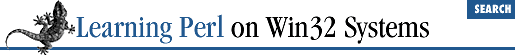home | O'Reilly's CD bookshelfs | FreeBSD | Linux | Cisco | Cisco Exam

### 2.2 Numbers

Although a scalar is either a number or a string,[ 1 ] consider numbers and strings separately for the moment. Numbers first, strings in a minute...

 A scalar can also be a reference, but that is an advanced topic.

### 2.2.1 All Numbers Use the Same Format Internally

As you'll see in the next few paragraphs, you can specify both integers (whole numbers, like 17 or 342) and floating-point numbers (real numbers with decimal points, like 3.14, or 1.35 times 10 25 ). But internally, Perl computes only with double-precision floating-point values.[ 2 ] This means that there are no integer values internal to Perl; an integer constant in the program is treated as the equivalent floating-point value.[ 3 ] You probably won't notice the conversion (or care much), but you should stop looking for integer operations (as opposed to floating-point operations), because there aren't any.

 A "double-precision floating-point value" is whatever the C compiler that compiled Perl used for a ``` double``` declaration.

 Unless you use "integer mode," but that is not the default.

### 2.2.2 Float Literals

A literal is the way a value is represented in the text of the Perl program. You could also call this a constant in your program, but we'll use the term literal. Literals are the way in which data is represented in the source code of your program as input to the Perl compiler. (Data that is read from or written to files is treated similarly, but not identically.)

Perl accepts the complete set of floating-point literals available to C programmers. Numbers with and without decimal points are allowed (including an optional plus or minus prefix), as well as tacking on a power-of-10 indicator ( exponential notation) with ``` E``` notation (that's ``` e``` or ``` E``` ). For example:

```1.25      # about 1 and a quarter
7.25e45   # 7.25 times 10 to the 45th power (a big number)
-6.5e24   # negative 6.5 times 10 to the 24th
# (a "big" negative number)
-12e-24   # negative 12 times 10 to the -24th
# (a very small negative number)
-1.2E-23  # another way to say that```

### 2.2.3 Integer Literals

Integer literals are also straightforward, as in:

```12
15
-2004
3485```

Don't start the number with a 0, because Perl supports octal and hexadecimal (hex) literals. Octal numbers start with a leading 0, and hex numbers start with a leading ``` 0x``` or ``` OX``` .[ 4 ] The hex digits A through F (in either case) represent the conventional digit values of 10 through 15. For example:

 The "leading zero" indicator works only for literals, not for automatic string-to-number conversion. You can convert a data string that looks like an octal or hex value into a number with ``` oct()``` or ``` hex()``` .

```0377  # 377 octal, same as 255 decimal
-0xff # negative FF hex, same as -255 decimal```2.1 What Is Scalar Data?2.3 Strings